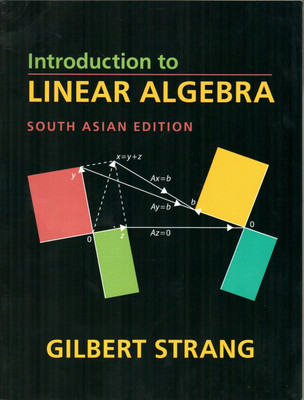## Thursday, July 30, 2015

### Introduction to Linear Algebra, Fourth Edition by Gilbert StrangGilbert Strang's textbooks have changed the entire approach to learning linear algebra -- away from abstract vector spaces to specific examples of the four fundamental subspaces: the column space and nullspace of A and A'.
Introduction to Linear Algebra, Fourth Edition includes challenge problems to complement the review problems that have been highly praised in previous editions. The basic course is followed by seven applications: differential equations, engineering, graph theory, statistics, fourier methods and the FFT, linear programming, and computer graphics.

Thousands of teachers in colleges and universities and now high schools are using this book, which truly explains this crucial subject.

Chapter 1: Introduction to Vectors; Chapter 2: Solving Linear Equations; Chapter 3: Vector Spaces and Subspaces; Chapter 4: Orthogonality; Chapter 5: Determinants; Chapter 6: Eigenvalues and Eigenvectors; Chapter 7: Linear Transformations; Chapter 8: Applications; Chapter 9: Numerical Linear Algebra; Chapter 10: Complex Vectors and Matrices; Solutions to Selected Exercises; Final Exam. Matrix Factorizations. Conceptual Questions for Review. Glossary: A Dictionary for Linear Algebra Index Teaching Codes Linear Algebra in a Nutshell.

Download